Function Repository Resource:

# EdgeBetweenQ

Quickly test for existence of an edge between two vertices

Contributed by: Michael Sollami
 ResourceFunction["EdgeBetweenQ"][g,e] yields True if e connects two vertices in the graph g and False otherwise.

## Details and Options

ResourceFunction["EdgeBetweenQ"] works on both Graph and EdgeTaggedGraph objects.
ResourceFunction["EdgeBetweenQ"] runs in time O(1).

## Examples

### Basic Examples (2)

Determine if two vertices are connected by any edge:

 In:=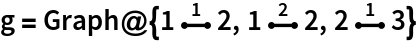Out=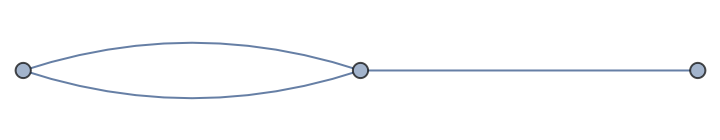In:=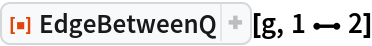Out=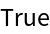Note that EdgeQ will give False because it takes edge properties, such as tags, into account:

 In:=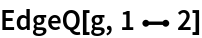Out=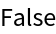### Scope (2)

EdgeBetweenQ works regardless of edge tag or edge type:

 In:=Out=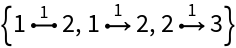In:=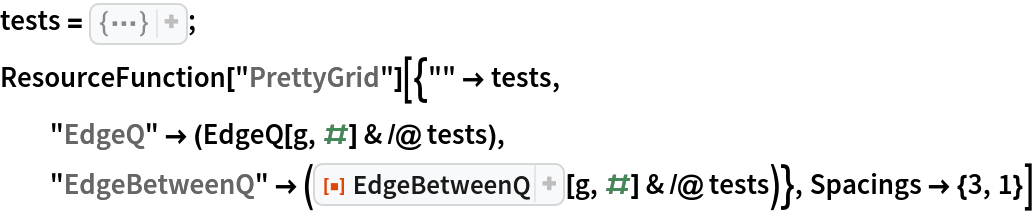Out=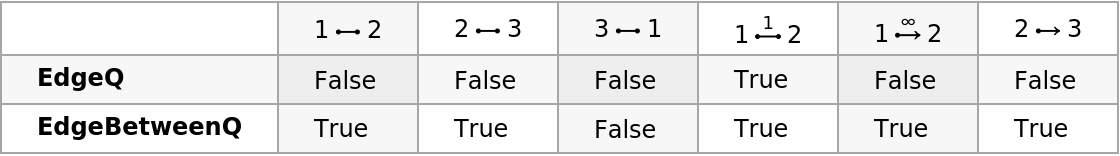Check whether an edge is part of a large edge-tagged graph:

 In:=Out=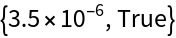Michael Sollami

## Version History

• 1.0.0 – 01 April 2020